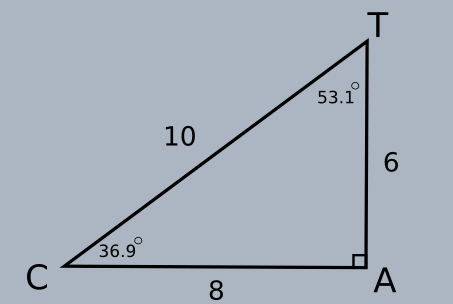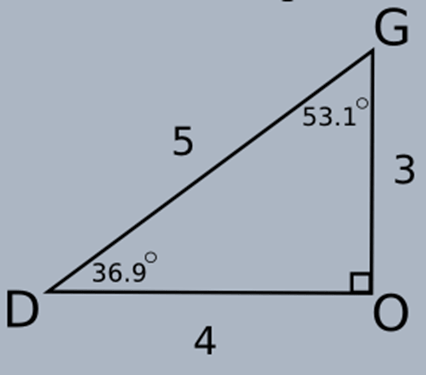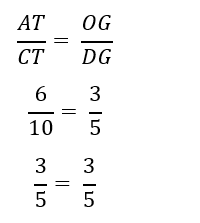# Trigonometric Proportions Worksheets

How to Evaluate Trigonometric Proportions? Sometimes learning trigonometric functions can become a tough job, especially if no one is able to understand its core concept. Nonetheless, resolving trigonometric functions deal with comparing similar right-angled triangles. In other words, if the triangles are proportional to each other, so will their corresponding angles. Let us look at the triangles below.If you look at the triangles above, the side AT corresponds to the side OG, while CT corresponds with DG. Now let us consider the sides of the fractions.You can now witness that the fractions are equal. This scenario will always occur in similar triangles.

• ### Basic Lesson

Guides students solving equations that involve an trigonometric proportions. Demonstrates answer checking. Identify True or False? 10 / 30 = 2 / 6 60 = 60 product of means and product of extremes are equal. So this is True.

• ### Intermediate Lesson

Demonstrates how to solve more difficult problems. x/2 = 6/8; solve for x.

• ### Independent Practice 1

A really great activity for allowing students to understand the concept of triangle proportions.

• ### Independent Practice 2

Students find the value of trigonometric proportions in assorted problems. The answers can be found below.

• ### Homework Worksheet

Students are provided with problems to achieve the concepts of the trigonometric proportions.

• ### Skill Quiz

This tests the students ability to evaluate math statements with the trigonometric proportions.